Home > CCA2 > Chapter 8 > Lesson 8.2.3 > Problem8-105

8-105.
1. Make a sketch of a graph p(x) so that p(x) = 0 would have the indicated number and type of solutions. Homework Help ✎

1. 5 real solutions

2. 3 real and 2 complex

3. 4 complex

4. 4 complex and 2 real

5. For parts (a) through (d), what is the lowest degree each function could have?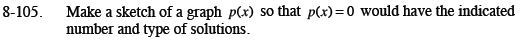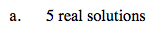Your graph should have five x-intercepts.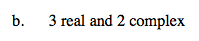Draw a graph with three x-intercepts that when shifted vertically would have five x-intercepts.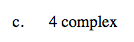Draw a graph with no x-intercepts. But, when it is shifted down it should have four x-intercepts.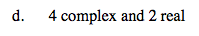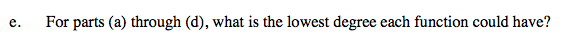The degree needs to be at least as high as the number of solutions.